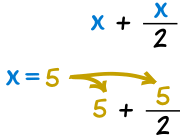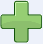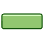# 代数――代换

"代" 的意思是把一样东西换为另外一样东西。

## 代换若：
 x − 2x=6 …………便可以把 6 "代入" x
 6 − 2 = 4

### 例子：若 x=2，10/x + 4 是多少？

10/2 + 4 = 5 + 4 = 9### 例子：若 x=5，x + x/2 是多少？

5 + 5/2 = 5 + 2.5 = 7.5

### 例子：若 x=3 及 y=4，x2 + xy 是多少？

32 + 3×4 = 3×3 + 12 = 21

32 + 3y = 9 + 3y

（只能做到这里了）

## 负数

### 例子：若 x = −2，1 − x + x2 是多少？

1 − (−2) + (−2)2 = 1 + 2 + 4 = 7

• − (−2) 变成 +2
• (−2)2 变成 +4

规则 加或   乘或 减 除相同的正负号变成 正号 3+(+2) = 3 + 2 = 5 3 × 2 = 6 6−(−3) = 6 + 3 = 9 (−3) × (−2) = 6不同的正负号变成 负号 7+(−2) = 7 − 2 = 5 3 × (−2) = −6 8−(+2) = 8 − 2 = 6 (−3) × 2 = −6